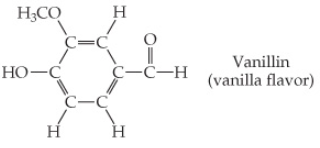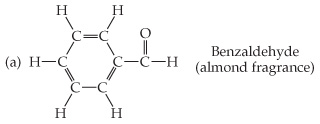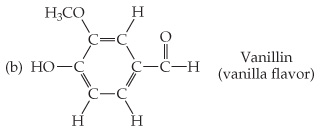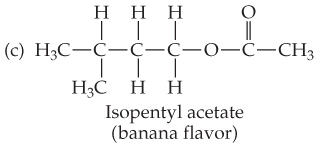# Problem: Based on the following structural formulas, calculate the percentage of carbon by mass present in each compound.Vanillin (vanilla flavor)

🤓 Based on our data, we think this question is relevant for Professor Veige's class at UF.

###### FREE Expert Solution

We are asked to calculate for the percent by mass (mass %) of C in vanillin(C8H8O3and to do that we’re going to use the mass percent formula shown below:

For the percent by mass C, we’re going to rewrite the equation as:###### Problem Details

Based on the following structural formulas, calculate the percentage of carbon by mass present in each compound.Vanillin (vanilla flavor)